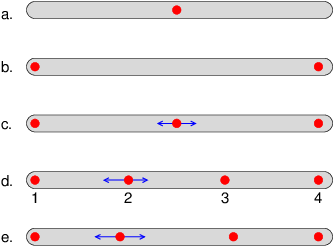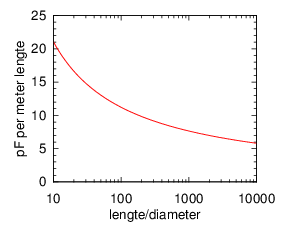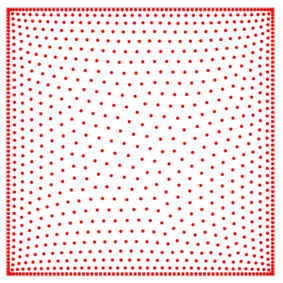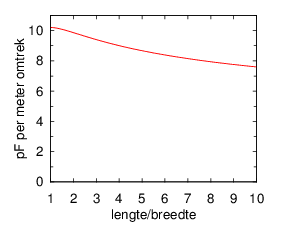# Capacitance of antenna elements

Pieter-Tjerk de Boer, PA3FWM pa3fwm@amsat.org

(This is an adapted version of part of an article that I wrote for the Dutch amateur radio magazine Electron, May 2014.)

While writing here about the MiniWhip antenna, I mentioned that in such active antennas the capacitance of the actual antenna element (a metal plate in the case of the MiniWhip, a metal wire or rod in case of an active whip antenna) is of importance, because it forms a capacitive voltage divider with the input capacitance of the amplifier connected to it. Also when building an input filter for such an antenna, as I discussed here, it is important to know the antenna capacitance. But that capacitance is not printed on it, so we have to calculate it. That is the topic of this article, with some theory but also a few handy rules of thumb.

## Capacitance

What is capacitance, actually? The capacitance of an object (a capacitor, or simply a piece of metal) is a measure for how easily one can store electric charge in or on it. There is a simple relationship between the amount of charge Q in a capacitor, the voltage U across that capacitor, and its capacitance C, namely Q = C × U. The voltage U is a measure of how much "effort" it takes to get that charge Q into that capacitance C: the smaller C is, at the same Q, the higher U needs to be. So in case of a small capacitor it takes more "effort", i.e., a higher voltage, to get that same charge into it.

Let's henceforth not consider the capacitance of a circuit component with two connection wires, but of a single loose piece of metal. The fact that it takes "effort" to put charge on it, is because the particles carrying that charge, the electrons, repell each other; bringing them close to each other takes effort, i.e., energy.

## Thin rodThe effect of the repulsion can be most easily illustrated using a thin rod (which at the same time is of course very relevant as an antenna form). See the figure.

First, we put one electron on the rod (figure a): that takes no effort because there is nothing exerting a repulsive force on that electron. Next, we put a second electron on the rod. Both electrons repell each other, causing them to move as far apart as possible, i.e. at the ends of the rod (figure b). If we add a third electron, it will sit right in the middle (figure c): that is where the repulsive forces (blue arrows) from the two electrons at the ends cancel each other.

Things get interesting with a fourth electron. One might expect that the four electrons would distribute themselves evenly over the rod, as sketched in figure d, but that is not correct. Just think about it: electron number 2 is repelled equally by electrons 1 and 3, so these two forces cancel each other, but there is also the a repelling force from electron 4, pushing it to the left. So electron 2 must move to the left, to a place where it is repelled more by electron 1 and less by the other two electrons; thus the forces balance and the electron can stay at that place. See figure e. (Note that electron 1 is pushed to the left by all of the other electrons; the only reason it doesn't move to the left, is simply that the conductive rod ends there, so the electron can't move further to the left.)We can of course continue this reasoning to much larger numbers of electrons, and in principle that leads to a calculation of the capacitance (namely the square of the charge divided by the energy stored in the electrons' repulsion).

For a thin rod, amateurs often use the rule of thumb "10 pF per meter of length". That is only an approximation. The precise value also depends on the ratio between length and thickness of the rod, as the graph shows. (See wikipedia and J.D. Jackson: Charge density on thin straight wire revisited, 2000.) For example, for a one meter long rod, with a diameter between 1.5 and 14 mm, the rule of thumb is accurate to within 20%.

## Rectangular plateAlso for a square piece of metal one can calculate how the electrons distribute themselves, such that for each electron the repulsive forces are in equilibrium. With 1000 electrons, this leads to this picture. We see again that the charge is not distributed evenly over the plate, but tends to concentrate near the edges. The cause for this is the same as with the metal rod, where the electrons were inclined to sit at the ends of the rod: they repell each other, and at the ends they can't move further.

In my technical notes on the MiniWhip I already wrote that I couldn't find a formula for the capacitance of such a square or rectangle. Since then, I did find a large number of scientific papers about calculating this capacitance using a computer, ranging from 1957 (D.K. Reitan, T.J. Higgins: Accurate Determination of the Capacitance of a Thin Rectangular Plate) to the current millenium (Jen-Tsai Kuo, Ke-Ying Su: Analytical Evaluation of the MoM Matrix Elements for the Capacitance of a Charged Plate, 2002). The calculation methods used here do not address individual electrons as done in the above picture, even though that would be possible, but they partition the square into smaller squares and then calculate as if within such a small square the charge is distributed evenly. From such calculations it follows that a square of 1 by 1 meter has a capacitance of 40.8 pF; if the square is bigger or smaller, this changes directly proportional to the length of the sides of the square (which is not directly proportional to its area).Using this method, I calculated the capacitance of a number of rectangles, leading to the adjacent figure. Vertically it shows the capacitance per meter of circumference, and horizontally the ratio between the plate's length l and width b. For l/b=1 we have a square; a square of 1 by 1 meter has, as noted above, a capacitance of 40.8 pF, and a circumference of 4 m, so the graph there shows 40.8/4=10.2 pF/m. For other rectangles we see that the capacitance per meter of circumference is lower. As an example, consider a MiniWhip with say a 35 by 80 mm plate: its length/width ratio is 2.3, and according to the graph that means 9.7 pF/m; the circumference is 0.23 m, so the capacitance is 2.2 pF. An easy rule of thumb is 10 pF per meter of circumference; in the above example, this gives an error of just 3%.

## Circle

For the capacitance of a circular plate, there is a precise formula: 4 ε0 = 35.42 pF per meter of diameter; again, 10 pF per meter of circumference is a decent approximation.

## Comparison

The fact that the number of pF per meter of circumference of a metal plate is approximately the same as the number of pF per meter of length of a thin rod, is not a coincidence. Recall that in the picture we saw that most of the charge on a metal plate sits near its circumference. Therefore, we can chop a large hole in the middle of such a plate without affecting the capacitance much. What remains resembles a rod along the circumference of the original plate; hence the similar number of pF per meter.

B.t.w., this means that a MiniWhip should work almost equally well if we wouldn't use a metal plate for the reception element, but a thick wire bent in the shape of that plate. Anyone want to give it a try?

## Sphere

The pictures in the above show that in case of a rod, there's a bit more charge at the ends than in the middle, and that in the case of a metal plate, this effect is much stronger. This is because in such a 2-dimensional plate there is more "space" along the edge: the edge is an entire line, rather than just two points as in the case of the rod. In case of a 3-dimensional object, this effect becomes even stronger: there all charges will sit at the edge, i.e., the surface.

For a sphere, physicists calculated that the capacitance is 4πε0 times the diameter; this boils down to 1.112 pF per cm of radius.

In old literature, capacitances are often not expressed in pF, but in cm. This is a consequence of the system of units that was in use back then, in which the unit of capacitance actually was the cm: a capacitance of 1 cm is the capacitance of a sphere with a 1 cm radius. This happens to be nearly 1 pF, so we can read the old literature as if 1 cm ≈ 1 pF.

## Parallel plates

So far, this article is about the capacitance of a loose piece of metal. One could consider this as a capacitor with that piece of metal as one connection and an infinitely large sphere, infinitely far away around it as the other connection.

However, practical capacitors always consist of two plates near each other, and in fact there's a well-known formula for the capacitance of two parallel metal plates in air: C=ε0A/d, where A is the area of the plates and d their distance. We see that in this case the capacitance is proportional to the area of the plates, and not to their circumference as in the case of a single metal plate. This difference is caused by the presence of that second plate. Both plates have charges of opposite sign; the positive charge on one plate attracts the negative charge on the other plate. If both plates are very close, this effect is stronger than the mutual repulsion which in the case of a single plate causes the charge to sit mostly at the edge of the plate. As a consequence, in a parallel-plate capacitor the charge distributes itself evenly over the surface, and thus the entire surface contributes to the capacitance.

Still, even in such a capacitor it remains true that if we double all sizes (i.e., length, width and height), the capacitance also only doubles: although the area A quadruples, the distance d doubles, so A/d doubles. This holds in general: if one doubles all sizes of any structure, the structure's capacitance also doubles.

shape precise capacitance rule of thumb
wire or thin rod 11.2 pF per meter of length if length/diam.=100
see figure for other cases
10 pF per meter of length
circular plate 35.42 pF per meter of diameter
i.e., 11.27 pF per meter of circumference
10 pF per meter of circumference
rectangular plate 40.8 pF for a 1m by 1m square
see figure for other cases
10 pF per meter of circumference
sphere 55.6 pF per m diameter 1 pF per cm radius